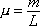For string (or thread or rope or cable or a rod but discussed in terms of string here) of a single kind of material with a constant cross-section, the linear density (mass-per-length) mu is equal to the mass of an amount of the string divided by the length L of that same amount of string.

Tip: In standing waves problems you are often faced with the mass of a sample of string and two lengths, the length of the entire sample of string and the length of that part of the string in which a standing wave exists. The question is: which length should you use in calculating the linear mass density? The answer is, the length of the entire sample. The mass and the length used in mu = m/L must both be for one and the same amount of string. Since you have the mass of the entire sample, you must use the length of the entire sample.